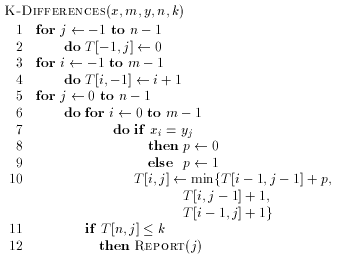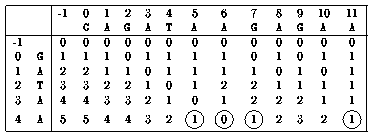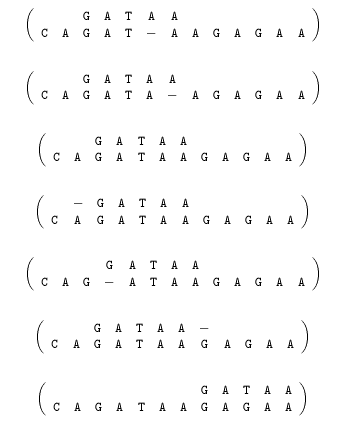Next: Longest common subsequences Up: Sequence comparison Previous: Levenshtein distance

# Approximate string matching with k differences

If we are interested in finding all the substrings of y which are at a distance less or equal to a given value k of x then it is enough to initialize all the values of the first line of the table with 0 (this means that the cost of insertions of letters of y at the beginning of x is null). The solutions are then given by all the values of the last row of T which are less or equal to k. This problem is know as approximate string matching with k differences.Example:

x = GATAA and y = CAGATAAGAGAA and k = 1Which gives the seven following alignments:Next: Longest common subsequences Up: Sequence comparison Previous: Levenshtein distance

e-mails: {Christian.Charras, Thierry.Lecroq}@laposte.net
Thu Feb 19 10:23:29 WET 1998